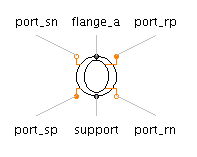First Order Hold - MapleSim Help

First Order Hold

First-order hold of a sampled-data systemDescription The First Order Hold component samples the input value, $u$, for a given sampling time and extrapolates the output value, $y$, through the values of the last two sampled input signals. The Signal Size parameter assigns the dimension of the input and output connectors.Connections

 Name Description Modelica ID $u$ Real input vector u $y$ Real output vector yParameters

 Name Default Units Description Modelica ID Sample Period $0.1$ $s$ Sample period of component samplePeriod ${T}_{0}$ $0$ $s$ First sample time instant startTime Signal Size $1$ Dimension of input and output signals signalSizeModelica Standard Library The component described in this topic is from the Modelica Standard Library. To view the original documentation, which includes author and copyright information, click here.